## Friday, January 26, 2007

### Questions on Centre of Mass

Two spheres of masses m1 and m2 (m1>m2) respectively are tied to the ends of a light, inextensible string which passes over a light frictionless pulley. When the masses are released from their initial state of rest, the acceleration of their centre of mass is
(a) [(m1–m2)/(m1+m2)]g
(b) [(m1–m2)/2(m1+m2)]g
(c) [(m1–m2)/4(m1+m2)]g
(d) [(m1–m2)/(m1+m2)]2g
(e) [4(m1–m2)/(m1+m2)]g
If r1 and r2 are the position vectors of the centres of the spheres, the position vector of their centre of mass is given by R = (m1r1 + m2r2)/(m1+m2). The acceleration of the centre of mass is therefore given by
a = d2R/dt2 = (m1d2r1/dt2 + m2 d2r2/dt2)/(m1+m2).
But d2r1/dt2 and d2r2/dt2, which are the accelerations of the masses m1 and m2 have the same magnitude (m1–m2)g/(m1+m2). If we take the acceleration of m1 (which is downwards) as positive, that of m2 is negative.
Therefore, a = [m1(m1–m2)g/(m1+m2) – m2(m1–m2)g/(m1+m2)] /(m1+m2).
On simplifying, this yields a = [(m1–m2)/(m1+m2)]2g. [Option (d)].
The following MCQ has been popular among question setters:
In the HCl molecule, the separation between the nuclei of the two atoms is about 1.27 Ǻ. The approximate distance of the centre of mass (from the hydrogen atom) of the HCl molecule, assuming the chlorine atom to be about 35.5 times as massive as the hydrogen atom is
(a) 0.27 Ǻ
(b) 0.56 Ǻ
(c) 1 Ǻ
(d) 1.24 Ǻ
(e) 2.26 Ǻ
The centre of mass of this two particle system is on the line joining the two atoms and in between these atoms. If ‘x’ is the distance of the centre of mass from the hydrogen atom, we have x = (1×0 + 35.5×1.27)/ (1+35.5) = 1.24 Ǻ, approximately.
Note that the equation giving the value of ‘x’ is the usual equation for the position vector of the centre of mass: R = (m1r1 + m2r2)/(m1+m2). We have taken the origin to be at the centre of the hydrogen atom so that r1 = 0 and r2 = 1.27 Ǻ.
[You can easily find the centre of mass of two particle systems by equating the ‘moments’ of the masses about the centre of mass. In the present case, 1×x = 35.5(1.27–x) from which x = 1.24 Ǻ].
Now consider the following simple question:
A proton and an electron, initially at rest, are allowed to move under their mutal attractive force. Their centre of mass will
(a) move towards the proton
(b) move towards the electron
(c) remain stationary
(d) move in an unpredictable manner.
The correct option is (c) because you require an external force to move the centre of mass of a system of particles. The mutual force of electrical attraction is an internal force which can not affect the position of the centre of mass of the system.

## Tuesday, January 23, 2007

### Two more Questions on Spherical Mirrors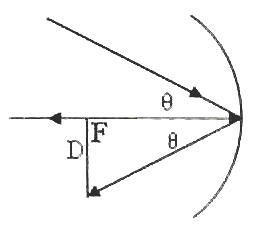The following question is quite simple, but many among you will find it confusing:
The sun subtends an angle of 0.5° on the earth’s surface. What is the diameter of the image of the sun, produced by a concave mirror of radius of curvature one metre?(a) 8.8mm (b) 6.4mm (c) 5mm (d) 4.4mm (e) data insufficient for arriving at an answer
In the figure θ is the angle subtended by the sun at the pole of the mirror. The real image of the sun is obtained at the focus of the mirror and this image also subtends the same angle θ at the pole of the mirror. Since the real image of the sun is obtained at the focus of the mirror, we have D/50 = 0.5×π/180 where D is the diameter of the image of the sun. [Note that the focal length of the mirror is 50cm and we have converted the angle of 0.5° in to radian].
From the above equation we obtain D = 0.44 cm (approximately) = 4.4mm.
The following question also is simple, but be careful while applying the signs in accordance with the Cartesian convention.
An erect image three times the size of a real object is formed with a concave mirror of radius of curvature 36cm. The distance of the object from the mirror is
(a) 24cm (b) 20cm (c) 16cm (d) 12cm (e) 8cm
Evidently, the signs of ‘f’ and ‘u’ are negative. Since the image is erect, it must be formed behind the mirror (virtual image) and the sign of ‘v’ is positive. Further, the image is magnified 3 times so that v=3u. Substituting these in the equation, 1/f = 1/u + 1/v, we have –1/18 = –1/u +1/3u from which u =12 cm.

## Monday, January 22, 2007

### Two Questions on Spherical Mirrors

Multiple Choice Questions on Spherical Mirrors
The following question pertains to the formation of a real image when a real object is kept in front of a concave mirror:
A bright rod of length 12 cm lies along the axis (parallel to the axis) of a concave mirror of radius of curvature 30 cm, the nearer end of the rod being at 30 cm from the mirror. The size of the image of the rod will be
(a) 20cm (b) 30.5cm (c) 40cm (d) 50.4cm (e) 60cm
The law of distances for a mirror is 1/f = 1/u + 1/v.
According to the Cartesian sign convention (see the post dated 22nd November 2006), the sign of the focal length (f) of a concave mirror is negative. The sign of the object distance ‘u’ also is negative since it has to be measured (from the pole), opposite to the direction of propagation of the incident ray.
Since the radius of curvature is 30cm, the focal length is 15cm. The image of the nearer end of the rod will be at a distance ‘v’ given by
– 1/15 = – 1/18 + 1/v. Note that we are not bothered about the sign of ‘v’ since it is an unknown quantity whose sign has to come out from the solution. We obtain v = – 90cm. The negative sign just means that this distance is measured opposite to the direction of propagation of the incident ray.
The distance of the image of the farther end of the rod can be obtained by putting u = – 30cm. In this case you will obtain the image distance as –30cm. [You can get it immediately without writing any equation if you remember that in the cse of a real object at 2f, the image is real and it will coincide with the object].
So, the size of the image is 90 – 30 = 60cm.
[Since you know that a concave mirror will form a real image when a real object is placed at a distance more than the focal length, u,v and f are all negative so that you could have worked out the above problem by treating all quantities as positive. But this method can be adopted only in special situations. Treating the known quantities with their proper signs is always the fool proof method].
Now, consider the following question which appeared in Kerala Engineering 2003 question paper:
An infinitely long rod lies along the axis of a concave mirror of focal length ‘f’. The nearer end is at a distance x > f from the mirror. Then the length of the image of the rod is
(a) f2/(x+f) (b) f2 /x (c) xf/(x–f) (d) xf/(x+f) (e) f2 /(x–f)
We have 1/f = 1/u + 1/v. The focal length ‘f’ and the object distance u = x are negative. Since ‘v’ is unknown we don’t bother about its sign and write, –1/f = –1/x + 1/v and obtain v = fx/(f–x). [This is a negative quantity since f is less than x as given in the question]. This gives the distance of the image of the nearer end of the rod. The distance of the image of the farther end of the rod is obtained by putting u = ∞ in the above equation. You will get the image distance as – f.
The length of the image of the rod is – f – [fx/(f–x)] = –f2 /(f–x) = f2 /(x–f).

## Friday, January 19, 2007

### Questions on Electrostatics

Charges +q, +q, –q and –q coulomb are arranged at the corners of an imaginary rectangle of sides 6m and 4m, as shown in the adjoining figure. A and B are the mid points of the longer sides. The potential difference between A and B is (in volts)
(a) 2.4×106q (b) 4.8×106q (c) 2.4×109q (d) 4.8×109q (e)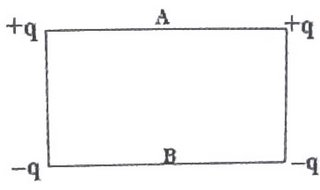9.6×109q
The potential at A due to the charges +q and +q is 2×(1/4πε0)(q/3) and the potential at the same point due to the charges –q and –q is 2×(1/4πε0))(–q/5). The resultant potential at A is 2×(1/4πε0)[(q/3)–(q/5)] = 2×(1/4πε0) (2q/15)= (1/4πε0) (4q/15).
Similarly, the resultant potential at B is 2×(1/4πε0)[(q/5) – (q/3)] =
2×(1/4πε0) (–2q/15) = (1/4πε0)(–4q/15).
The potential difference between points A and B is (1/4πε0))[(4q/15) – (–4q/15)] = (1/4πε0)×(8q/15). You should remember that 1/4πε0= 9×109. Therefore, the P.D. is 9×109×(8/15)q = 4.8×109q volts.
The following question appeared in Punjab CEET 2003 question paper:
As shown in figure, if the point C is earthed and the point A is given a potential of 2000 volt, then the potential at B will be
(a) 400 V (b) 500 V (c) 1000 V (d) 1300 V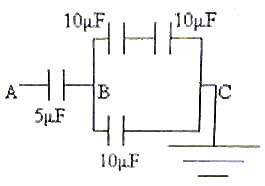The effective capacitance between the points B and C is 15 μF because 10μF and 10μF in series in the upper branch make 5μF and this is in parallel with 10μF in the lower branch. So, in effect 2000 V is applied across the series combination of 5μF and 15μF connected between A and C. Since the potential difference across capacitors connected in series is inversely proportional to the capacitance, the applied P.D. of 2000 volts get divided in the ratio 3: 1 across 5μF and 15μF [1500 V : 500V]. The potential at B with respect to the grounded point C is therefore 500V.
Now consider the following question which appeared in IIT 2002 question paper:
Two identical capacitors have the same capacitance C. One of them is charged to potential V1 and the other to potential V2. The negative ends of the capacitors are connected together. When the positive ends are also connected, the decrease in energy of the combined system is
(a) ¼ C(V12 – V22) (b) ¼ C(V12 + V22) (c) ¼ C(V1 – V2)2 (d) ¼ C(V1 + V2)2
The initial energy of the system is ½ CV12 + ½ CV22 = ½ C(V12+V22).
On connecting the capacitors (in parallel), the net capacitance of the system is 2C and the net voltage across the combination is ½ (V1+V2). [The total charge is C(V1+V2) so that the net voltage is C(V1+V2)/ 2C = (V1+V2)/2].
Therefore, final energy of the system is(½) × 2C[(V1+V2)/2]2 = ¼ C(V12+V22 +2V1V2).
On subtracting the final energy from the initial energy, we obtain the decrease in energy as ¼ C(V1–V2)2, given by option (c).
You will find more multiple choice questions on Electrostatics with solution in the post dated 9th October 2006.

## Thursday, January 11, 2007

### Multiple Choice Questions on Wave Optics

Consider the following MCQ which is meant for gauging your grasp of the principle of superposition of waves:Two laser beams of the same wave length and intensities 9I and I are superposed. The minimum and maximum intensities of the resultant beam are:
(a) 8I and 10I (b) 0 and 10I (c) 4I and 16I (d) 0 and 16I (e) 4I and 10I
Since the intensity is directly proportional to the square of the amplitude, the minimum and maximum intensities are[√(9I) – √I]2 and [√(9I) + √I]2 respectively, which are [2√I]2 and [4√I]2. So, the correct option is 4I and 16I.
Diffraction at single slit deserves your special attention since questions involving it are often seen in Medical and Engineering entrance test papers. Here is a question which appeared in Kerala Engineering Entrance 2006 question paper:
The width of a single slit if the first minimum is observed at an angle 2º with a light of wave length 6980 Ǻ is
(a) 0.2 mm (b) 2×10–5 mm (c) 2×105 mm (d) 2 mm (e) 0.02 mm

The angular position of the first minimum is λ/a. Therefore, λ/a = 2×(π/180), on converting the angle in degrees to the angle in radian. This gives a = 180 λ/2π
= 180×6980×10–10/2π = 0.02×10–3 m = 0.02 mm.
Here is a question which may easily mislead you:
In a double slit experiment, the separation between the slits is 1 mm and the distance between the double slit and the screen is 1m. If the slits are illuminated by monochromatic light of wave length 6000 Ǻ, what is the separation between the 2nd dark bands on either side of the central band?
(a) 0.9 mm (b) 1.2 mm (c) 1.8 mm (d) 3 mm (e) 4.2 mm
As you know, the central band in Young’s double slit experiment is bright. The distance from the centre of this bright band to the centre of the first dark band (on one side) is half a band (fringe) width. The distance from the centre of the first dark band to the centre of the second dark band is one band width. So, the distance between the centre of the central band and the second dark band on one side is one and a half times the band width. The distance between the second dark bands on either side is therefore three times the band width.
Now, band width, β = λD/d where λ is the wave length of light used, D is the distance of the screen from the double slit and ‘d’ is the separation between the two slits. The answer to the question is 3β = 3 λD/d =3×6000×10–10×1/(1×10–3) = 1.8×10–3 m = 1.8 mm.
The following MCQ appeared in AIEEE 2004 question paper:The maximum number of possible interference maxima for slit separation equal to twice the wave length in Young’s double slit experiment is
(a) infinite (b) five (c) three (d) zero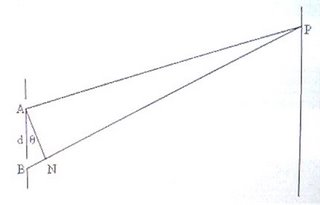If ‘d’ is the slit separation (AB in fig.), the path difference between the waves reaching a point P on the screen is BP – AP = dsinθ and the condition to produce the nth maximum (bright fringe) at P is
d sinθ = nλ = nd/2.
Therefore, n = 2 sinθ. Since ‘n’ must be an integer, the allowed values are 2,1,0, –1 and –2 because the values of sinθ lie between +1 and –1. So, there can be, at the most, 5 bands only (including the central band.
Now, consider the following MCQ involving interference of light reflected from a thin film:
A transparent film of refractive index 1.5 is viewed in reflected monochromatic light of wave length 6000 Ǻ. If the angle of refraction in to the film is 60º, what is the smallest thickness of the film to make it appear dark?
(a) 4×10–4 mm (b) 5×10–4 mm (c) 6×10–4 mm (d) 7×10–4 mm (e) 8×10–4 mm
In the reflected system, the condition for darkness is 2μtcosr = nλ where μ is the refractive index, ‘t’ is the thickness (of the film), ‘r’ is the angle of refraction in to the film, ‘n’ is the order of the interference band and λ is the wave length of the light used.
Since the minimum thickness is required, n =1 so that 2μtcosr = λ from which t = λ/2μcosr = 6000×10–10/(2×1.5×0.5) = 4×10–7m = 4×10–4 mm.
(Note that the condition for darkness in the reflected system is 2μtcosr = nλ [and not (2n+1)λ/2] because of the phase change of π (equivalent to a path change λ/2) when reflection occurs at a denser medium for light traveling through a rarer medium).
The following MCQ which appeared in IIT 2001 entrance test paper is worth noting:
Two beams of light having intnsities I and 4I interfere to produce a fringe pattern on a screen. The phase difference between the beams is π/2 at point A and π at point B. Then the difference between the resultant intensities at A and B is
(a) 2I (b) 4I (c) 5I (d) 7I
Since the wave amplitudes are added vectorially to produce the resultant intensity at any point, the resultant intensity at A = I + 4I + 2√I×√(4I)cos(π/2) = 5I. [Note that the amplitude is directly proportional to the square root of intensity].
Similarly, resultant intensity at B = I + 4I + 2√I×√(4I)cosπ = I.
The difference between the rresultant intensities is 5I–I = 4I.
Now consider the following question which involves the measurement of refractive index using interference fringe shift:
When a thin sheet of transparent material of thickness 4×10–3 mm is placed in the path of one of the interfering beams in Young’s double slit experiment, it is found that the central bright fringe shifts through a distance equal to four fringes. What is the refractive index of the transparent material? (Wave length of light used is 5893 Ǻ).
(a) 1.378 (b) 1.432 (c) 1.523 (d) 1.546 (e) 1.589
Before introducing the transparent sheet, the path difference between the beams arriving at the centre of the fringe system is zero. When the sheet of thickness ‘t’ is introduced with its plane normal to one of the beams, a path difference equal to μt – t = (μ–1)t is produced. [Note that a path length ‘t’ in a medium of refractive index μ is equivalent to a path length μt in air because of the shrinkage of the waves].
Therefore we have (μ–1)t = nλ so that μ = (nλ/t) +1 = (4×5893×10–10)/(4×10–6) + 1 = 1.5893.

## Monday, January 01, 2007

### Additional Multiple Choice Questions on Simple Harmonic Motion

The following post is in continuation of the post ‘Multiple Choice Questions on Simple Harmonic Motion’, dated 19th October 2006:
Let us consider the following MCQ which appeared in Kerala Engineering Entrance 2005 question paper:
A particle starts SHM from its mean position. Its amplitude is ‘a’ and total energy is ‘E’. At one instant its kinetic energy is 3E/4. Its displacement at that instant is
(a) a/√2 (b) a/2 (c) a/√(3/2) (d) a/√3 (e) a
The equation of the simple harmonic motion is y = A sinωt with usual notations. The total energy is (½) m ω2a2 and the kinetic energy is (½)m ω2(a2 –y2).
Therefore we have (½) m ω2a2 = E and
(½)m ω2( a2 –y2) = 3E/4.
Dividing the second equation by the first, 1 – y2/a2 = ¾, from which y = a/2.
The following question will be interesting to you: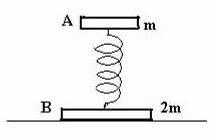Two discs A and B of masses m and 2m respectively are connected by a light spring and placed on a table (Fig.) so that the disc B is on the table and the disc A is resting on the spring. If the frequency of oscillation of the spring on slightly depressing and releasing the disc A, is ‘f’, the frequency of oscillation on suddenly removing the table is (a) f/2 (b) f/√2 (c) f (d) √(2/3) f (e) √(3/2) f
When disc B is resting on the table, disc A functions as the oscillating mass in a spring-mass system and the period of oscillation, as usual, is given by T = 2π√(m/k) where ‘k’ is the spring constant. The frequency of oscillation is f = (1/2π)√(k/m).
When the table is removed, the system falls freely and both discs are free to oscillate. The reduced mass (μ) of the vibrating system is given by μ = m×2m/(m+2m) = (2/3)m.
The frequency of vibration in this case is obtained by replacing ‘m’ in the above expression for frequency by (2/3)m so that the new frequency is (1/2π)√[k/(2/3)m] = (1/2π)√(k/m) ×√(3/2) = f ×√(3/2) [Option (e)].
Now, consider the following question:
The kinetic energy and potential energy of a simple harmonic motion (of amplitude ‘A’ ) will be equal when the displacement is
(a) A/√2 (b) A√2 (c) A/2 (d) A/3 (e) A/√3
Equating the kinetic energy to the potential energy, we have ½ m ω2( A2 –y2) = ½ m ω2y2, from which ( A2 –y2) = y2. Therefore, y = A/√2.
Consider the following MCQ:
Two light springs of force constants k1 and k2, loaded with equal masses undergo vertical oscillations. If the maximum velocities of the masses are the same, the ratio of the amplitudes of oscillations is
(a) k1 /k2 (b) 1 (c) k1 2 /k2 2 (d) k2 /k1 (e) √(k2 /k1 )
If A1 and A2 are the amplitudes of oscillations of the first and the second system respectively and ω1 and ω2 their angular frequencies, we have ω1 A1 = ω2 A2 , since the maximum velocities are equal. Therefore, A1 /A2 = ω21 .
But ω = √(k/m) for linear simple harmonic motion so that A1 /A2 = √(k2 /m2 )/ √(k1 /m1 ) = √(k2 /k1 ) since the masses are equal.
[Even if you don’t remember the relation, ω = √(k/m), you can work out this since the maximum potential energy (and maximum kinetic energy) of an oscillating spring is ½kA2 . Since the maximum velocities are equal (as specified in the question), the maximum kinetic energies are equal so that we have, ½k1 A1 2 =½k2 A2 2 , from which A1 /A2 = √(k2 /k1 )].